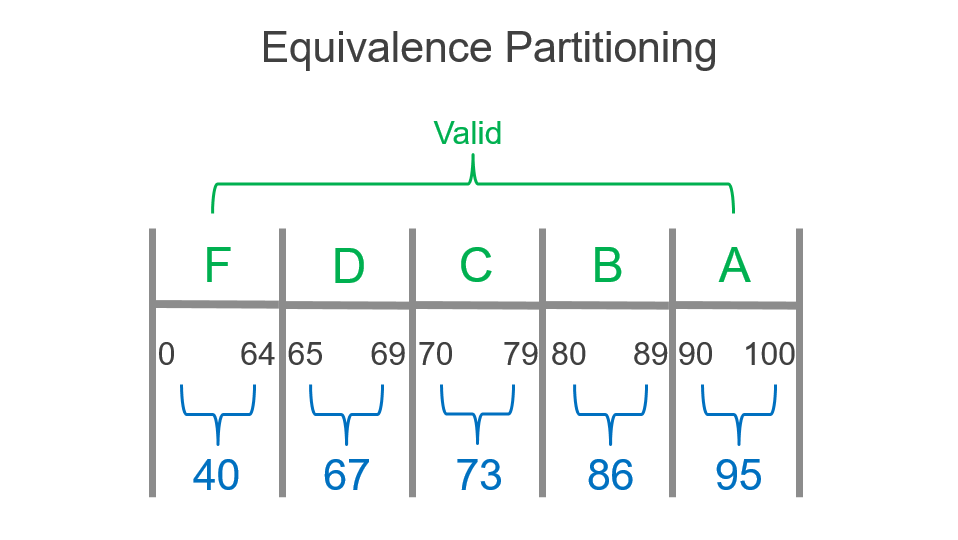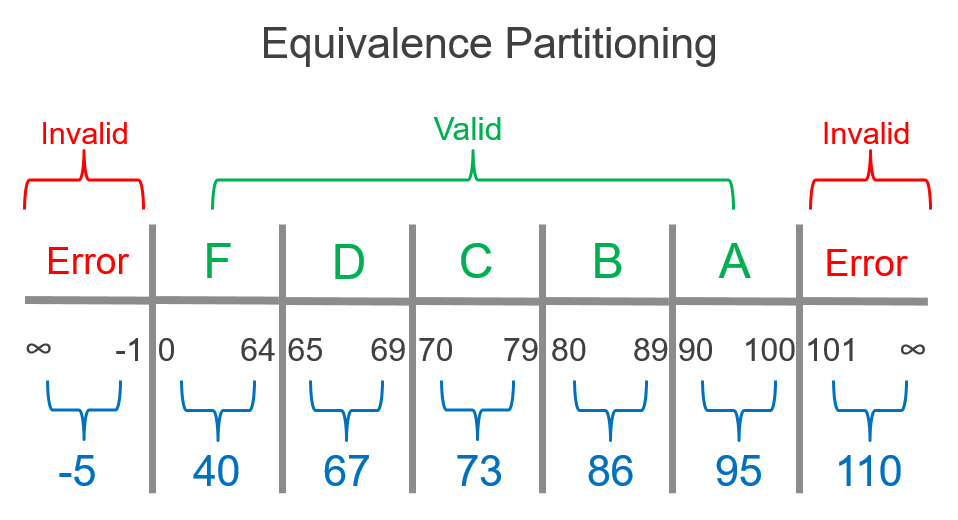# Test Design Techniques: Equivalence Partitioning

Posted in Functional Testing

The test case can be considered the backbone of software testing. It is the set of instructions and inputs that is followed by a software tester with the goal of finding instances where the outcome was not expected - a bug or defect in the software.

Well-written test cases contain scenarios likely to uncover bugs and defects, so it is crucial that the scenarios are valid for proper test coverage. How do we design our tests so that they capable of making sure the software is thoroughly tested or useful in uncovering defects? Thankfully, there are helpful test design techniques that can be followed that do exactly that. By employing these techniques when designing test cases, testers can utilize test data that's most effective at triggering these bugs and defects.

Equivalence partitioning (EP) is a good technique to use first in test design. With EP, you divide the range of input data into groups (called equivalence partitions or equivalence classes) that can be considered the same.

With this assumption, we only test one piece of test data from each partition because we assume every other piece of test data within that partition is going to be treated the same by the software. We assume the opposite is true as well – if one piece of test data from the partition fails, we assume the other data in the partition fail as well. This is a useful assumption because it lets us use a minimal number of test scenarios instead of potentially dozens or hundreds of test cases.

Let's look at an example to visualize this. Imagine you are testing gradebook software used by teachers, checking that a student's number grade on a test is given the correct letter grade upon being input into the system. 0 to 64 should output F, 65 to 69 should output D, and so on.We can easily see our valid partitions, grouped by the ranges that make up the letter grade. The idea is that we'll choose a single input from each partition to use in our testing, since we're assuming that all numbers in a partition will behave the same, and testing every possible number in these ranges would be too time-consuming.

For F we'll use 40, 67 for D, 73 for C, 86 for B, and 95 for A. The particular numbers in a partition do not matter. 95 is as good as 92, which is as good as 98 - all of these should be expected to give you the letter grade A.What if the teacher accidentally inputs a negative number or a number above 100? We should test those invalid partitions as well to make sure the system behaves correctly, for example checking that an error message displays.

Our invalid partition at one of the spectrum can cover an infinite range of negative numbers, so we'll just choose -5. On the other end of the spectrum, we'll choose 110 to check for numbers greater than 100. So, our test cases based on EP would use these seven values, the minimum number of numeric inputs to cover every expected behavior of the application.Test Case #Input Value Expected Output
1-5Error
240F
367 D
473 C
586 B
695A
7110Error

COMING SOON: Part 2 of the Test Design Technique series will cover the importance of using boundary value analysis alongside equivalence partitioning.